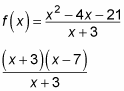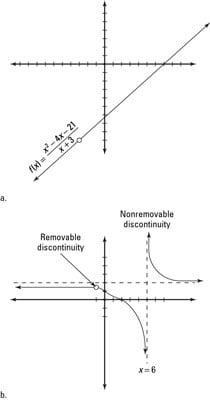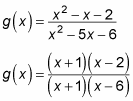##### Pre-Calculus For DummiesAs your pre-calculus teacher will tell you, functions that aren't continuous at an x value either have a removable discontinuity (a hole in the graph of the function) or a nonremovable discontinuity (such as a jump or an asymptote in the graph):
• If the function factors and the bottom term cancels, the discontinuity at the x-value for which the denominator was zero is removable, so the graph has a hole in it.

For example, this function factors as shown:After canceling, it leaves you with x – 7. Therefore x + 3 = 0 (or x = –3) is a removable discontinuity — the graph has a hole, like you see in Figure a.The graph of a removable discontinuity leaves you feeling empty, whereas a graph of a nonremovable discontinuity leaves you feeling jumpy.
• If a term doesn't cancel, the discontinuity at this x value corresponding to this term for which the denominator is zero is nonremovable, and the graph has a vertical asymptote.

The following function factors as shown:Because the x + 1 cancels, you have a removable discontinuity at x = –1 (you'd see a hole in the graph there, not an asymptote). But the x – 6 didn't cancel in the denominator, so you have a nonremovable discontinuity at x = 6. This discontinuity creates a vertical asymptote in the graph at x = 6. Figure b shows the graph of g(x).